# What is Conservative Force

• Conservative forces
A force is called conservative when all the work along any closed path is zero.

Or:
The work that a conservative force
at a mass point in the displacement
performed from point 1 to point 2, is independent of the path on which the shift takes place.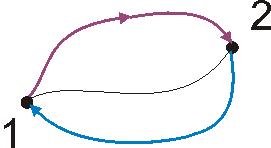• Under the energy a system is understood to be its ability to do work.
• Potential energy
In a conservative force field, the change in the potential energy of a system is equal to the work done on the system by an external force. The potential energy is fixed up to a constant.

Examples:

Altitude energy:

Tension energy of a spring: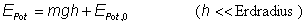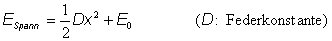A conservative force has the tendency to accelerate a particle in a direction in which the potential energy becomes smaller.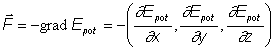;
• Kinetic energy, kinetic energy
The kinetic energy of a body of mass m amounts to: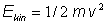• A closed system has no interaction and no exchange of matter with its surroundings.
• Theorem of conservation of mechanical energy.
In a closed system in which only conservative forces (no frictional forces) act, the mechanical energy remains constant (sum of potential and kinetic energy).
• The Power P indicates how much energy per unit of time is transferred from one system to another: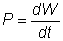If a force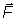is exerted on a particle that moves with the speed.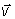moves, the following applies: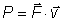;